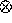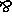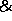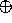Abstracts of Linear Logic Papers

Making Logic Programs Reactive

James Harland and Michael Winikoff

Proceedings of the JICSLP'98 Workshop on Transactions and Change in Logic Databases (Dynamics'98) 43-58, Manchester, June, 1998.

Logic programming languages based on linear logic have been of recent interest, particularly as such languages provide a logical basis for programs which execute within a dynamic environment. Most of these languages are implemented using standard resolution or backward-chaining techniques. However, there are applications for which the use of forward-chaining techniques within a dynamic environment are appropriate, such as genetic algorithms, active databases and agent-based systems, and for which it is difficult or impossible to specify an appropriate goal in advance. In this paper we discuss the foundations for a forward-chaining approach (or in logic programming parlance, a bottom-up approach) to the execution of linear logic programs, which thus provides forward-chaining within a dynamic environment. In this way it is possible not only to execute programs in a forward-chaining manner, but also to combine forward- and backward-chaining execution. We describe and discuss the appropriate inference rules for such a system, the formal results about such rules, the role of search strategies, and applications. Paper

Strategies for Logic Programming Languages

Tatjana Lutovac and James Harland

Proceedings of the CADE14 Workshop on Strategies in Automated Deduction Townsville, July, 1997.

Logic programs consist of formulas of mathematical logic and various proof-theoretic techniques can be used to design and analyse execution models for such programs. We briefly review the main problems, which are questions that are still elusive in the design of logic programming languages, from a proof-theoretic point of view. Existing strategies which lead to the various languages are all rather sophisticated and involve complex manipulations of proofs. All are designed for analysis on paper by a human and many of them are ripe for automation. We aim to perform the automation of some aspects of strategies for logic programming language, in order to assist in the design of these languages. In this paper we describe the first steps towards the design of such an automatic analysis tool. We investigate the usage of particular proof manipulations for the analysis of logic programming strategies. We propose a more precise specification of sequent calculi inference rules that we use as a framework for the generalisation and automation-oriented specification of the permutation process. Paper

Towards the Automation of the Design of Logic Programming Languages

Tatjana Lutovac and James Harland

Technical Report 97-30, Department of Computer Science, RMIT, April,1997.

Logic programs consist of formulas of mathematical logic and various proof-theoretic techniques can be used to design and analyse execution models for such programs. We briefly review the main problems, which are questions that are still elusive in the design of logic programming languages, from a proof-theoretic point of view. Existing approaches and analyses which lead to the various languages are all rather sophisticated and involve complex manipulations of proofs. All are designed for analysis on paper by a human and many of them are ripe for automation. We aim to perform the automation of some aspects of proof-theoretic analyses, in order to assist in the design of logic programming languages. In this paper we describe the first steps towards the design of such an automatic analysis tool. We propose a more precise specification of sequent calculi inference rules that we use for the generalisation and automation-oriented specification of permutation process. We illustrate different problems and proposed solutions through the examples in various formulations of sequent calculi for classical, intuitionistic, linear, modal, minimal logic, including single-conclusioned and multiple-conclusioned systems. Paper

Resource-Distribution via Boolean Constraints (Extended Abstract)

James Harland and David Pym

to appear in the Proceedings of the Fourteenth International Conference on Automated Deduction (CADE-14), Townsville, July, 1997.

Proof-search (the basis of logic programming) with multiplicative inference rules, such as linear logic'sR andL, is problematic because of the required non-deterministic splitting of resources. Similarly, searching with additive rules such asL andR requires a non-deterministic choice between two formulae. Many strategies which resolve such non-determinism, either locally or globally, are available.

We present a characterization of a range of strategies for distributing and selecting resources in linear sequent calculus proof-search via a sequent calculus annotated with Boolean constraints. Strategies are characterized by calculations of solutions of sets of Boolean equations generated by searches. Our characterization encompasses lazy (or local), eager (or global) and intermediate (mixed local and global) strategies. Paper

Programming in Lygon: An Overview

James Harland, David Pym and Michael Winikoff

Proceedings of the Fifth International Conference on Algebraic Methodology and Software Technology 391-405, Munich, July, 1996.

Recently, there has been much interest in the derivation of logic programming languages based on linear logic, a logic of resource-consumption. Such languages provide a notion of resource-oriented programming, often leading to programs that are more elegant and concise than their equivalents in languages, such as Prolog, based on classical logics. We discuss, with examples, the design, implementation and applications of Lygon, a linear logic programming language derived from a proof-theoretic analysis of which occurrences of the linear connectives provide an adequate basis for programming. In common with other linear logic programming languages, Lygon allows clauses to be used exactly once in a computation, thereby avoiding the need for the explicit resource-counting often necessary in Prolog-like languages. Just as linear logic is a strict extension of classical logic, Lygon is a strict extension of (pure) Prolog: all (pure) Prolog programs can be executed by the Lygon system. Hence all the features of classical pure logic programs are available in Lygon, together with new ones based on linear logic. These include global variables, a theoretically transparent notion of state, mutual exclusion operators and various constructs for manipulating clauses. All of these follow from the basis of Lygon in linear logic and do not require extra-logical features for their definition. Paper

Some Applications of the Linear Logic Programming Language Lygon

Michael Winikoff and James Harland

Proceedings of the Nineteenth Australasian Computer Science Conference 262-271, Melbourne, January, 1996.

We describe and discuss the applications of a logic programming language called Lygon. This language is based on linear logic, a logic designed with bounded resources in mind. Linear logic may be thought of as a generalisation of classical logic, and as a result Lygon contains various features which do not exist in (pure) Prolog, whilst maintaining all the features of (pure) Prolog. In this paper we describe various applications of this language, which include graph problems, and problems involving states and actions. In logic programming languages based on classical logic, it is possible to write elegant solutions for problems over acyclic graphs. By making use of properties of linear logic, it is possible to write similarly elegant solutions for cyclic graphs as well. As linear logic enables changes of state to be neatly expressed, it is straightforward to give Lygon solutions to problems such as the Yale shooting problem and the blocks world, and we give example solutions to each. Paper

Implementing the Linear Logic Programming Language Lygon

Michael Winikoff and James Harland

Proceedings of the International Logic Programming Symposium 66-80, Portland, Decemeber, 1995.

There has been considerable work aimed at enhancing the expressiveness of logic programming languages. To this end logics other than classical first order logic have been considered, including intuitionistic, relevant, temporal, modal and linear logic. Girard's linear logic has formed the basis of a number of logic programming languages. These languages are successful in enhancing the expressiveness of (pure) Prolog and have been shown to provide natural solutions to problems involving concurrency, natural language processing, database processing and various resource oriented problems. One of the richer linear logic programming languages is Lygon. In this paper we investigate the implementation of Lygon. Two significant problems that arise are the division of resources between sub-branches of the proof and the selection of the formula to be decomposed. We present solutions to both of these problems. Paper

Implementation and Development Issues for the Linear Logic Programming Language Lygon

Michael Winikoff and James Harland

Proceedings of the Eighteenth Australasian Computer Science Conference 563-572, Adelaide, February, 1995.

We describe and discuss the implementation of a new logic programming language called Lygon. This language is based on linear logic, a logic designed with bounded resources in mind. Linear logic may be thought of as a generalisation of classical logic, and as a result Lygon contains various features which do not exist in (pure) Prolog, whilst maintaining all the features of (pure) Prolog. In this paper we describe the implementation of this language, which posed a variety of programming challenges. The operational model for the language is based on the notion of goal-directed provability, a notion which has been much studied in the literature. However, there is a significant amount of non-determinism in this notion of proof. Hence the task of developing a systematic and deterministic manner in which to search for proofs requires some intricate and novel implementation techniques. We describe and discuss our particular solution, as well as the features of the language and its applications. Paper

A Uniform Proof-Theoretic Investigation of Linear Logic Programming

David Pym and James Harland

Journal of Logic and Computation 4:2:175-207, April, 1994.

In this paper we consider the problem of identifying logic programming languages for linear logic. Our analysis builds on a notion of goal-directed provability, characterized by the so-called uniform proofs, previously introduced for minimal and intuitionistic logic. A class of uniform proofs in linear logic is identified by an analysis of the permutability of inferences in the linear sequent calculus. We show that this class of proofs is complete (for logical consequence) for a certain (quite large) fragment of linear logic, which thus forms a logic programming language. We obtain a notion of resolution proof, in which only one left rule, of clause-directed resolution, is required. We also consider a translation, resembling those of Girard, of the hereditary Harrop fragment of intuitionistic logic into our framework. We show that goal-directed provability is preserved under this translation. Paper

A Note on the Implementation and Applications of Linear Logic Programming Languages

James Harland and David Pym

Proceedings of the Seventeenth Annual Computer Science Conference 647-658, Christchurch, January, 1994.

Traditional logic programming languages, such as Prolog, have their origins in classical logic. Recently it has been shown how logic programming languages can be based on linear logic, a logic designed with bounded resources in mind. In this paper we discuss the implementation issues for the linear logic programming language Lygon, and describe some of the novel features of the language. These include global variables, a notion of state, mutual exclusion operators and constructs for the manipulation of clauses. We also show how a bin packing problem can be solved simply and elegantly in Lygon. Paper

On Resolution in Fragments of Classical Linear Logic

James Harland and David Pym

Proceedings of the Russian Conference on Logic Programming and Automated Reasoning 30-41, St. Petersburg, July, 1992. Published as Lecture Notes in Artificial Intelligence 624, Springer-Verlag, 1992.

We present a proof-theoretic foundation for logic programming in Girard's linear logic. We exploit the permutability properties of two-sided linear sequent calculus to identify appropriate notions of uniform proof, definite formula, goal formula, clause and resolution proof for fragments of linear logic. The analysis of this paper extends earlier work by the present authors to include negative occurrences of par and positive occurrences of ! (of course !) and ? (why not ?). These connectives introduce considerable difficulty. We consider briefly some of the issues related to the mechanical implementation of our resolution proofs. Paper

The Uniform Proof-Theoretic Foundation of Linear Logic Programming (Extended Abstract)

James Harland and David Pym

Proceedings of the International Logic Programming Symposium 304-318, San Diego, October, 1991.

We present a proof-theoretic analysis of a natural notion of logic programming for Girard's linear logic. This analysis enables us to identify a suitable notion of uniform proof. This in turn enables us to identify choices of classes of definite and goal formulae for which uniform proofs are complete and so to obtain the appropriate formulation of resolution proof for such choices. Resolution proofs in linear logic are somewhat difficult to define. This difficulty arises from the need to decompose definite formulae into a form suitable for the use of the linear resolution rule, a rule which requires the selected clause to be deleted after use, and from the presence of the modality ! (of course). We consider a translation --- resembling those of Girard --- of the intuitionistic hereditary Harrop formulae and intuitionistic uniform proofs into our framework, and show that certain properties are preserved under this translation. We sketch the design of an interpreter for linear logic programs. Paper